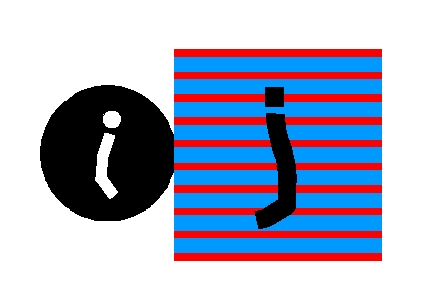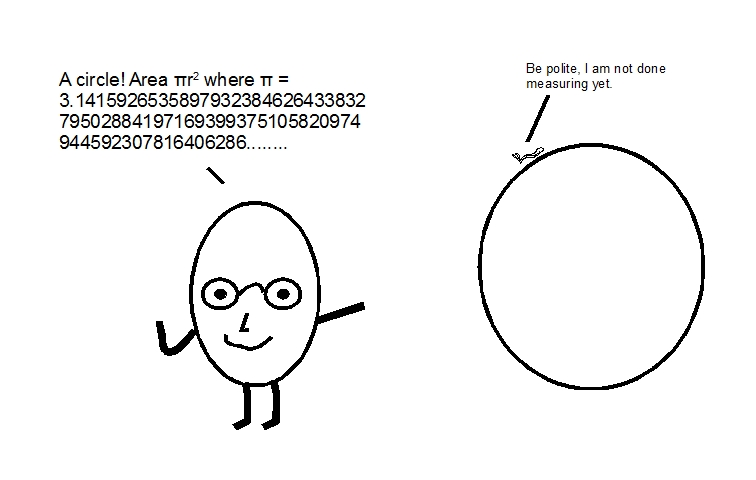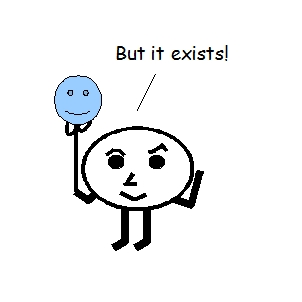The Probability Abacus
 Home SUMMARY: We will describe how to count in the discrete measurement space in terms of P(E) = 1 events. Our Abacus counts only P(E) = 1 events.What we have been trying to do is to describe a given system without having to use the traditional coordinate frames, such as Cartesian, Spherical, or Cylindrical.  We suspect that origin is not accurately measurable due to the observer's limitations.  And therefore we introduced a discrete measurement space based on an observer's capacity. One may also think of it as an introduction of a minimum quantum (observer1-observer2), whose value is based on the maximum of the observer's capacity.  What does this quantum mean we do not know yet.       We have established the origin in the discrete measurement space, we called j-space*.  Then we should be very specific how the count is progressed from the origin or “zero”, based on an established criterion.  We define the criterion for progression of the count as P(E) = 1 or PE1 event, where P(E) = 1 implies that the probability P(E) of an event E taking place, is equal to 1.  Only when a PE1 measurement is completed by an observer we should progress the count to next integer, i.e. "0" a PE1 event, is incremented to "1" which has to be another PE1 event.  We can not increment the count from a PE1 event to a PE<1 event.         Therefore we have two important considerations before the description of a system is developed, first we have to define the conditions leading to establishment of the origin and then we have to increment the count based on PE1 events only**.            Remember that our origin is infinite and a PE1 event, therefore the next PE1 event also has to be infinite.  We can think of universe as an ongoing measurement which is yet to be completed.The Bug Measures a Circle                Within the discrete measurement space we have to appreciate the importance of a PE1 measurement.  An example of such case will be the determination of a circle by two observers of different capacities, one is a bug constrained to crawl on the circumference of the circle and making measurements and other is a human.       In the case of an unlikely event the bug may have completed all the measurements, i.e. all the outcomes are determined, a PE1 event is completed, and the bug can develop an algorithm based on its measurements to interpret the result, whatever it could be for the bug.  The human at the other hand knows that it is a circle and draws conclusions from it without making measurements.We note that the human observer does not apply a measurement force to make a determination of circle whereas bug has to. This is an important consideration for an observer to be efficient, that is, "an efficient observer does not apply a measurement force to make a measurement or an observation". This criterion leads to optimum use of the resources while making a measurement, an optimization which is a fundamental requirement in nature.        We have another concern.  What if the circle being measured, is drawn infinitesimally thin?  Then neither bug nor human observer will be able to measure it at their existing resolutions and therefore they will both arrive at the same conclusion that the circle does not exist, but circle does exist.Therefore a PE1 event has taken place but it is determined to be non-existent because of the measurement limitations, represented by observer's inability to determine the origin with absolute accuracy.  The possibility that a PE1 event has occurred but can not be measured due to observer's limitations is quite worrisome. Measured Numbers           We are going to make out description more specific to the observer's capacity.  We have already mentioned that observers with with different capacities will be measuring the values of  0's,  ∞'s and the intervals [ 0, ∞ ] differently.  We will attach a subscript to the numbers to signify the measurement space specific to the observer's capacity.       For example an observer who can measure "null" will have infinite capacity.  We will call such observer Obsi (i for infinite) and we denote the interval measured by Obsi as [ 0i, ∞i ].  Similarly we denote the observer with finite capacity as Obsj and the interval measured by Obsj as [ 0j, ∞j ].  We have to keep in mind that Obsj represents a pair of observers.       It is important to note that numbers 0j, 1j, 2j,.........,∞j  represent the "measured numbers".  The measured numbers will represent a picture of less or more details for an observer of capacity greater or less respectively than that of Obsj,because the definitions of  origin will be different for each observer.  At the same time 0j will be measured as 0j and 1j will be measured as 1j and so on, by the observers of identical capabilities.  We will get used to these notations.      In order to measure , an observer requires infinite capacity which is equivalent to saying that the observer of finite capacity will have to measure an infinite information space, where the term "information" represents an abstract notion we will try to resolve.  We further represent this infinite information space by a "true-point".  A true-point can be thought of as a disc without a boundary, an abstract region whose characteristics can not be measured.  We will need a quantum toy based on the particle in a box analogy to play with this concept. ______________________ * i-space represents an infinite information space, an infinite source, or an exact source.  ** For example, we can think of second universe only when we have completely measured the first one, a PE1 event.   We keep in mind that natural numbers begin with "1". The concepts of "0" and negative numbers apply only when the time-axis has been initiated due to entropy.Information on www.ijspace.org is licensed under a Creative Commons Attribution 4.0 International License. 1 orange consists of billions of cells, 1 cell consists of billions of atoms, 1 atom contains billions of elementary states......., 1 universe contains billions of oranges, 1 source contains billions of universes, Have we counted "one" yet? - A limerick from the Bugworld, Unknown Author.